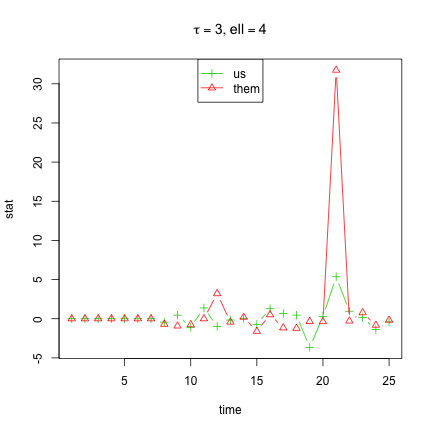# Anomaly Detection using Graph Embedding on Time Series of Charity Graphs

### JHU XDATA Team

Sun Jun 2 22:19:18 2013

## Build Time Series of Graphs, $$G_{donor\times charity,t}$$

Transactions are grouped in $$numt=25$$ equal time intervals, and a time series of graphs is built of length $$numt$$. For each time interval $$t$$, an edge exist between a donor and a charity at $$t$$ if there is a transaction between the two at that interval.

numt <- 25
ggg <- buildTSG(numt)

##  25

##  8052

##  756


## Build $$G_{donor}$$ (fixed throughout time)

The dissimilarities between donors are computed, and the adjacency matrix for the donor graph is randomly generated based on the random dot product graph model. Since the dissimilarities are constant, the graph is also constant throughout time.

Aj <- buildGj2(donor)

##  8052 8052


## Calc Stats on $$G_{charity,t}$$ using STFP

Given the constant graph of $$G_{donor}$$ and its embedding, we find embeddings of the time-dependent $$G_{donor\times charity,t}$$ for each $$t=1,\ldots,numt$$ . We then sample a random dot product graph based on the embedding and compute the local scan statistic at each time interval.

dmax <- 20
numt <- length(ggg)
us <- them <- rep(0, numt)
for (i in 1:numt) {
Atable <- get.incidence(ggg[[i]])
out <- inverse.rdpg.oos(Aj, dmax, Atable)
stfp <- as.matrix(out\$X.oos)
P <- cossim(stfp)
P <- exp(-P^2)
Aj2 <- rg.sample(P)
(us[i] <- max(local.scan.igraph(gj2, k = 1)))
(them[i] <- max(local.scan.igraph(gj2, k = 1, "them")))
}


## Plot Stats

Plot the time series of ''us'' vs ''them'' local statistics.## Maps

The points are the charities who are the $$(k=1)$$ neighbors of $$\arg\max_v(scan1)$$ at each time $$t$$. The size of the point is the total amount of donations to the charity.